## Example Questions

1 2 3 4 6 Next →

### Example Question #281 : Act Math

A kite has a side length of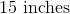and another side length of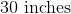. Find the perimeter of the kite.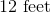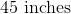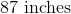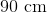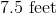Explanation:

By definition a kite must have two sets of equivalent sides. Since we know that this kite has a side length ofand another side with a length of, each of these two sides must have one equivalent side.

The perimeter of this kite can be found by applying the formula: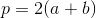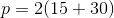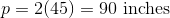Note, though, that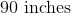does not appear as an answer choice. Thus, convertinto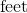by: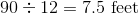### Example Question #13 : How To Find The Perimeter Of Kite

A kite has a side length of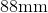and another side length of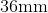. Find the perimeter of the kite.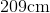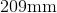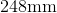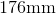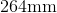Explanation:

a kite must have two sets of equivalent sides. Since we know that this kite has a side length ofand another side with a length of, each of these two sides must have one equivalent side.

The perimeter of this kite can be found by applying the formula: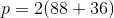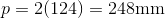Note: the correct solution can also be found by: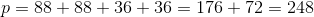### Example Question #291 : Geometry

A kite has a side length of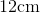and another side length of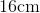. Find the perimeter of the kite.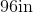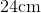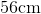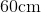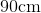Explanation:

A kite must have two sets of equivalent sides. Since we know that this kite has a side length ofand another side length of, each of these two sides must have one equivalent side.

The perimeter of this kite can be found by applying the formula: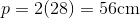### Example Question #1 : How To Find The Area Of A Kite

Find the area of a kite with the diagonal lengths of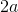and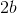.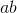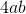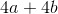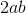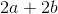Explanation:

Write the formula to find the area of a kite.  Substitute the diagonals and solve.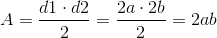### Example Question #332 : Advanced Geometry

Find the area of a kite with diagonal lengths of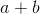and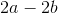.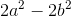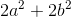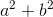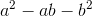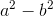Explanation:

Write the formula for the area of a kite.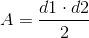Plug in the given diagonals.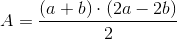Pull out a common factor of two inand simplify.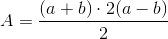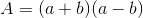Use the FOIL method to simplify.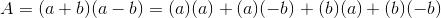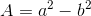### Example Question #1 : How To Find The Area Of A Kite

Find the area of a kite if one diagonal is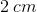long, and the other diagonal is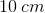long.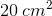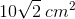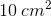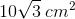Explanation:

The formula for the area of a kite is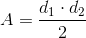Plug in the values for each of the diagonals and solve.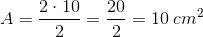1 2 3 4 6 Next →

### All ACT Math Resources